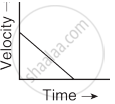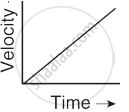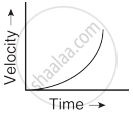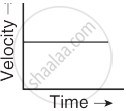Tamil Nadu Board of Secondary EducationSSLC (English Medium) Class 9th

# Match the Following. Sr. No. List I List II 1. Motion of a body covering equal distances in equal interval of time 2. Motion with non uniform acceleration 3. Constant retardation 4. Uniform accelerati - Science

Match the Columns

Match the Following.

 Sr. No. List I List II 1. Motion of a body covering equal distances in equal interval of time A.2. Motion with non uniform acceleration B.3. Constant retardation C.4. Uniform acceleration D.#### Solution

 Sr. No. List I List II 1. Motion of a body covering equal distances in equal interval of time D.2. Motion with non uniform acceleration C.3. Constant retardation A.4. Uniform acceleration B.Concept: Motion and Rest
Is there an error in this question or solution?